# Which Australian bank offers the best term deposits for 3 months05.02.2020

## Bankchart.com.au has surveyed the terms of term deposits for 3 months in the national currency. The survey covered all the deposits with any form of interests repayment, regardless of the replenishment option or the deposit partial withdrawal option. Our website's analysts have studied the loan offers from all Australian banks. Review date - 05.02.2020

As of 25 January 2020 the deposits under review have been offered by 33 banks.  The average interest rate under such deposits equals 1,30 % p.a. The total number of the deposits programs available for the specified terms is 83.

The maximal return among the deposits under review is offered by Bank of China. The interest rate under "TERM DEPOSIT" deposit program constitutes 2,05 % p.a. The table below shows the best offers by each bank selected by Bankchart.com.au (in terms of profitability) for the aforementioned terms.

### The TOP-33 term deposits for 3 months, as of 25 January 2020

#

Name

Interest Rate, %

1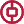Bank of China

«TERM DEPOSIT»

2,05

interest rate, %

rate type

fixed

interest periodicity

at maturity

minimum sum

\$ 500 000,00

2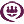RACQ

«Term Deposit»

1,75

interest rate, %

rate type

fixed

interest periodicity

quarterly

minimum sum

\$ 500,00

3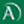Arab Bank

«Fixed Term Deposit»

1,70

interest rate, %

rate type

fixed

interest periodicity

at maturity

minimum sum

\$ 50 000,00

4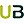Ubank

«Term deposit»

1,70

interest rate, %

rate type

variable

interest periodicity

at maturity

minimum sum

\$ 0,00

5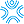Bank First

«Term Deposits»

1,65

interest rate, %

rate type

fixed

interest periodicity

monthly

minimum sum

\$ 50 000,00

6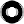Macquarie Bank

«Term Deposit»

1,65

interest rate, %

rate type

fixed

interest periodicity

monthly

minimum sum

\$ 0,00

7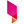Bank Australia

«Term Deposits»

1,65

interest rate, %

rate type

fixed

interest periodicity

at maturity

minimum sum

\$ 500,00

8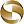Mega International Commercial Bank

«Term Deposits»

1,65

interest rate, %

rate type

fixed

interest periodicity

at maturity

minimum sum

\$ 1 000,00

9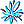AMP Bank

«Term Deposits»

1,65

interest rate, %

rate type

fixed

interest periodicity

at maturity

minimum sum

\$ 25 000,01

10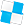Police Bank

«Term Deposits»

1,60

interest rate, %

rate type

fixed

interest periodicity

at maturity

minimum sum

\$ 1 000,00

11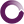Beyond Bank Australia

«Farm Management Deposit»

1,60

interest rate, %

rate type

fixed

interest periodicity

at maturity

minimum sum

\$ 200 000,00

12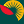Suncorp Bank

«Term Deposit»

1,55

interest rate, %

rate type

fixed

interest periodicity

at maturity

minimum sum

\$ 1 000 000,00

13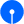State Bank of India

«Term Deposits»

1,55

interest rate, %

rate type

fixed

interest periodicity

at maturity

minimum sum

\$ 100,00

14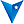BankVic

«Term deposit»

1,55

interest rate, %

rate type

fixed

interest periodicity

at maturity

minimum sum

\$ 25 000,00

15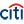Citibank

«Term deposit»

1,51

interest rate, %

rate type

fixed

interest periodicity

monthly

minimum sum

\$ 250 000,00

16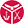Defence Bank

«Term Deposits»

1,50

interest rate, %

rate type

fixed

interest periodicity

at maturity

minimum sum

\$ 1 000,00

17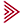Bendigo and Adelaide Bank

«Term deposit»

1,50

interest rate, %

rate type

fixed

interest periodicity

at maturity

minimum sum

\$ 5 000,00

18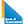Bank of Queensland

«Term deposit»

1,50

interest rate, %

rate type

fixed

interest periodicity

at maturity

minimum sum

\$ 5 000,00

19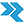Rural Bank

«Short Term Deposit»

1,50

interest rate, %

rate type

fixed

interest periodicity

monthly

minimum sum

\$ 5 000,00

20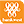Bank of Western Australia

«Online Term Deposit»

1,50

interest rate, %

rate type

fixed

interest periodicity

at maturity, annually

minimum sum

\$ 5 000,00

21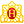Heritage Bank

«Term Deposits»

1,50

interest rate, %

rate type

fixed

interest periodicity

at maturity

minimum sum

\$ 1 000,00

22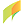G&C Mutual Bank

«Term Deposits»

1,50

interest rate, %

rate type

fixed

interest periodicity

at maturity, annually

minimum sum

\$ 1 000,00

23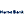Hume Bank

«Term Deposits»

1,35

interest rate, %

rate type

fixed

interest periodicity

at maturity, annually

minimum sum

\$ 5 000,00

24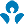Australia and New Zealand (ANZ)

1,35

interest rate, %

rate type

fixed

interest periodicity

at maturity

minimum sum

\$ 5 000,00

25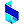Bank of Sydney

«Online Term Deposit»

1,35

interest rate, %

rate type

fixed

interest periodicity

at maturity

minimum sum

\$ 50 000,00

26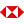HSBC Bank

«Term Deposits»

1,35

interest rate, %

rate type

fixed

interest periodicity

at maturity

minimum sum

\$ 5 000,00

27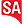Bank SA

«Term Deposits»

1,30

interest rate, %

rate type

fixed

interest periodicity

at maturity

minimum sum

\$ 1 000,00

28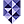Bank of Melbourne

«Term Deposits»

1,30

interest rate, %

rate type

fixed

interest periodicity

at maturity

minimum sum

\$ 1 000,00

29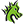St. George Bank

«Term Deposits»

1,30

interest rate, %

rate type

fixed

interest periodicity

at maturity

minimum sum

\$ 1 000,00

30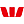Westpac Bank

«Term Deposit»

1,30

interest rate, %

rate type

fixed

interest periodicity

at maturity, annually

minimum sum

\$ 5 000,00

31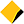Commonwealth Bank of Australia

«Term Deposits»

1,25

interest rate, %

rate type

fixed

interest periodicity

annually, every 6 months

minimum sum

\$ 49 999,00

32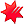National Australia Bank

«NAB Term Deposits»

1,25

interest rate, %

rate type

fixed

interest periodicity

at maturity

minimum sum

\$ 5 000,00

33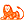ING Direct

«Personal Term Deposit»

1,10

interest rate, %

rate type

fixed

interest periodicity

at maturity

minimum sum

\$ 10 000,00

To view the up-to-date rating of the deposits at our website here.